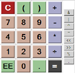Engineering ToolBox - Resources, Tools and Basic Information for Engineering and Design of Technical Applications!

# Scientific Calculator

## Online scientific calculator.

In the display window of the calculator below you write the complete mathematical expressions before calculation. This feature is very handy, especially when working with long complicated formulas. The values and expressions can be typed by using either keyboard or calculator buttons and the expressions can be reused and modified by accessing the history tab.

Note! - use radians for trigonometric functions.

Note that the calculator relies on the syntax of javascript. The constants and the syntax for the mathematical functions are explained below.

### Constants

• ans : the last calculated result
• PI : pi = 3.14159265...
• E : e = 2.71828182...
• LOG2E : log of e base 2
• LOG10E : log of e base 10
• LN2 : log of 2 base e
• LN10 : log of 10 base e
• SQRT2 : square root of 2
• SQRT1_2 : square root of 1/2

### Functions

• abs(a) : the absolute value of a
• acos(a) : arc cosine of a
• asin(a) : arc sine of a
• atan(a) : arc tangent of a
• atan2(a,b) : arc tangent of a/b
• ceil(a) : integer closest to a and not less than a
• cos(a) : cosine of a
• exp(a) : exponent of a
• floor(a) : integer closest to and not greater than a
• log(a) : log of a base e
• max(a,b) : the maximum of a and b
• min(a,b) : the minimum of a and b
• pow(a,b) : a to the power b
• random() : pseudorandom number in the range 0 to 1
• round(a) : integer closest to a
• sin(a) : sine of a
• sqrt(a) : square root of a
• tan(a) : tangent of a

### Examples

Some simple expressions:

• 23 = pow(2,3) = 8
• log(3) = 1.099
• 2 * (3 + 2) = 10

A more complicated formula for calculating the pressure drop in compressed air pipe lines (using same values as the link)

• dp = 7.57 q1.85 L 104 / d5 p = 7.57 * pow(10,1.85) * 100e4 / (pow(52.501,5) * 7) = 0.19

Note that the expressions can be written in any editor and transferred to the calculator display by using "copy and paste".### Display

This calculator can handle input numbers in several different bases:

• Decimal (Base 10): Numbers that do not start with a zero like 15 or 3.14e15. Decimal numbers can contain digits 0-9, decimals, and scientific notation.
• Hexadecimal (Base 16): Integers that start with a zero x like 0x1a5. Hexadecimal numbers can contain digits 0-9 and a-f (or A-F) but no decimal or scientific notation.
• Octal (Base 8): Integers that start with a zero like 073. Octal numbers can contain digits 0-7 but no decimal or scientific notation.
• Binary (Base 2): Integers that start with a zero b like 0b101. Binary numbers can contain digits 0 and 1 but no decimal or scientific notation.

^ is a bitwise xor operation. To raise a number to a power use pow() function.

### Thanks

to ostermiller.org for providing this calculator under the terms of the GNU General Public License as quoted below:

"This program is free software; you can redistribute it and/or modify it under the terms of the GNU General Public License as published by the Free Software Foundation; either version 2 of the License, or (at your option) any later version.

This program is distributed in the hope that it will be useful, but WITHOUT ANY WARRANTY; without even the implied warranty of MERCHANTABILITY or FITNESS FOR A PARTICULAR PURPOSE. See the GNU General Public License for more details.
"

## Related Topics

• Mathematics - Mathematical rules and laws - numbers, areas, volumes, exponents, trigonometric functions and more.

## Engineering ToolBox - SketchUp Extension - Online 3D modeling!

Add standard and customized parametric components - like flange beams, lumbers, piping, stairs and more - to your Sketchup model with the Engineering ToolBox - SketchUp Extension - enabled for use with the amazing, fun and free SketchUp Make and SketchUp Pro .Add the Engineering ToolBox extension to your SketchUp from the SketchUp Pro Sketchup Extension Warehouse!

Translate

## Privacy

We don't collect information from our users. Only emails and answers are saved in our archive. Cookies are only used in the browser to improve user experience.

Some of our calculators and applications let you save application data to your local computer. These applications will - due to browser restrictions - send data between your browser and our server. We don't save this data.

## Citation

• Engineering ToolBox, (2008). Scientific Calculator. [online] Available at: https://www.engineeringtoolbox.com/scientific-calculator-d_1302.html [Accessed Day Mo. Year].

Modify access date.

. .

#### Scientific Online Calculator3 30